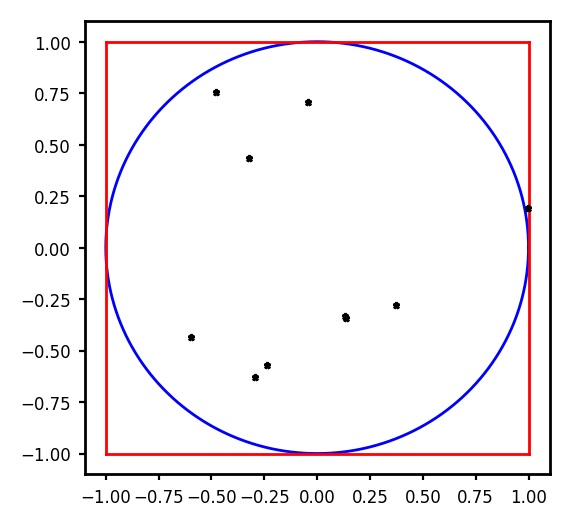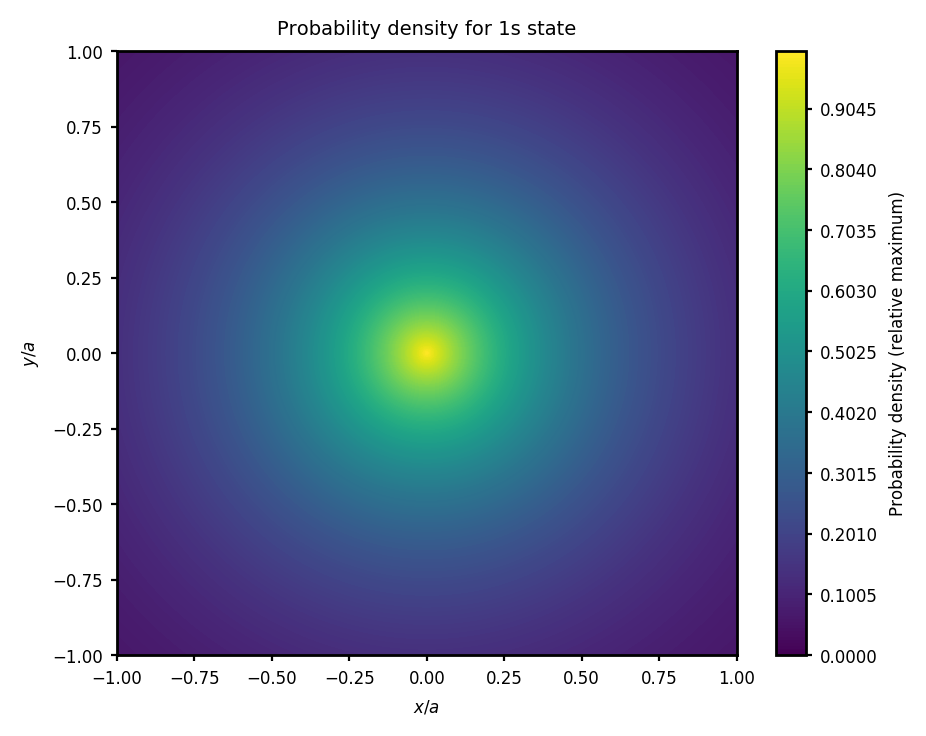# The Size of a Hydrogen Atom¶

### Example – Quantum Mechanics¶

Last edited: January 19th 2019

It is often said that the size of the hydrogen atom is two Bohr radii, or approximately 1 Å. However, the electron is not at a constant distance from the nucleus; all the information we can obtain from the Schrödinger equation, is the probability of finding the electron within an interval $r+\mathrm{d}r$ from the nucleus. It is given by

$${\rm d}P = |\psi_{nlm}|^2 \mathrm{d}r,$$

where $\psi_{nlm}$ is the respective hydrogen wave function. Therefore, it is interesting to calculate the probablility of finding the electron within one Bohr radius when the hydrogen atom is in the 1s (ground) state, and see in how far this represents the size of the atom. The required integral can be solved analytically, but in this notebook we will make use of Monte Carlo integration. Firstly, the integration method is introduced with an example.

### Monte Carlo Integration¶

Monte Carlo methods are statistical simulations which make use of sequences of random numbers. As an example, we can look at a circle with radius $r=1$, placed inside a square with sides of length 2. We can then generate two random numbers, $x$ and $y$, corresponding to a point $(x,y)$ inside the square. In the figure below we have plotted the square and circle, and generated 10 random points which are also included in the plot.

In :
%matplotlib inline
from __future__ import division
import numpy as np
import matplotlib.pyplot as plt
from numpy.random import uniform

# Set common figure parameters:
newparams = {'axes.titlesize': 7, 'axes.labelsize': 6,
'axes.linewidth': 1, 'savefig.dpi': 300,
'lines.linewidth': 1.0, 'lines.markersize': 2.4,
'figure.figsize': (3, 3), 'figure.subplot.wspace': 0.4,
'ytick.labelsize': 6, 'xtick.labelsize': 6,
'xtick.major.size': 2, 'ytick.major.size': 2,
'legend.handlelength': 1.5, 'figure.dpi': 200}
plt.rcParams.update(newparams)

#Plot circle
x = lambda theta: np.cos(theta)
y = lambda theta: np.sin(theta)
theta = np.linspace(0, 2*np.pi, 2000)
plt.plot(x(theta), y(theta), 'b')

#Plot square
plt.plot([-1,1],[-1,-1],'r',[-1,1],[1,1],'r',[-1,-1],[-1,1],'r',[1,1],[-1,1],'r')

plt.xlim([-1.1, 1.1])
plt.ylim([-1.1, 1.1])

#Generate two sets of 10 random numbers
x = uniform(-1, 1, 10)
y = uniform(-1, 1, 10)
plt.plot(x, y, '*k', lw=0.1);We see from the figure that many of the points are located inside the circle, and we can determine if they are inside by checking if $$\sqrt{x^2+y^2} \leq r.$$ For a large number of random points, $N$, it is reasonable to assume that the following relation holds $$\frac{n}{N}=\frac{V_\mathrm{circ}}{V_\mathrm{square}},$$ where $n$ is the number of points inside the circle, and $V_\mathrm{circ}$ and $V_\mathrm{square}$ is the "volume" (a surface in 2-D obviously) of the circle and square, respectively. To integrate a function $f(x,y)$ over the area of the circle, we simply add the function value at each point inside the circle, $$I =\frac{V_\mathrm{square}}{N} \left[ \sum_{n} f(x,y) \right].$$

Effectively, this represents a Riemann sum with control volumes $\Delta V=V_\mathrm{square}/N$ and a function $f(x,y)$ that is set to be identical to zero outside the circle.

As a short demonstration, we can integrate the function $f(x,y)=\sqrt{x^2+y^2}=r=f(r)$ over a circle of radius $r=1$. Use $N = 10^5$, and $V_\mathrm{square}=(2r)^2=4$.

In :
N = 1e6
V = 4
i = 0
n = 0
while i < N:
x = uniform(-1, 1)
y = uniform(-1, 1)
r = np.sqrt(x**2+y**2)
if r <= 1:
n = n + r
i = i + 1

I = V/N*n
print(r'Monte Carlo integral: %f' % I)
print(r'Analytical integral: %f' % (2/3*np.pi))

Monte Carlo integral: 2.093711
Analytical integral: 2.094395


As we see, the correspondence is quite good! The method described above can also be used in three and more dimensions.

Back to the hydrogen atom now...

### The Hydrogen Atom¶

The wavefunction of the 1s state of the hydrogen atom is

$$\psi_{100}(r) = \frac{1}{\sqrt{\pi a^3}}\exp(-r/a),$$

independent of $\theta$ and $\phi$, and where $a=0.529$ Å is the Bohr radius. The probability density is given by

$$\left|\psi_{100}(r)\right|^2 =\frac{1}{\pi a^3}\exp(-2r/a).$$

Using Monte Carlo integration, the integral of the probability density within a sphere of radius $a$, the Bohr radius, is calculated below. The analytical value is also computed.

In :
N = 1.0e5     # Number of random numbers
a = 0.529e-10 # Bohr radius
i = 0
n = 0.0
j = 1.0       # number of Bohr radii to integrate over
while i < N:
x = uniform(-j*a, j*a)
y = uniform(-j*a, j*a)
z = uniform(-j*a, j*a)
r = np.sqrt(x**2 + y**2 + z**2)
if r <= j*a:
n = n + np.exp(-2*r/a)/(np.pi*a**3)

i = i + 1

prob = n/N*(2*j*a)**3

print(r'The probability within one Bohr radius is: %s' % prob)
probAnalytical = 1-np.exp(-2*j)*(1+2*j+2*j**2)
print(r'The analytical probability is: %s' % probAnalytical)

The probability within one Bohr radius is: 0.324051965924
The analytical probability is: 0.323323583817


We see that there is again good agreement between the computed and analytical values.

It is rather surprising that there is only a probability of about $0.32$ of finding the electron within one Bohr radius and yet this is regarded as the size of a hydrogen atom! Below is a contour plot of the probability density for the 1s state, as seen in the $xy$-plane.

In :
p = 1000
xs = np.linspace(-j*a, j*a, p, True)
X,Y = np.meshgrid(xs, xs)
psi2 = np.zeros([p, p])

r = np.sqrt(X**2+Y**2)

psi2 = np.exp(-2*r/a)/(np.pi*a**3)

plt.figure(figsize=(5,4))
levels = np.linspace(0, 1, 200, True)
C = plt.contourf(X/a, Y/a, psi2*(np.pi*a**3), levels)
plt.title(r'Probability density for 1s state')
plt.ylabel(r'$y/a$')
plt.xlabel(r'$x/a$')
cbar = plt.colorbar(C)
cbar.ax.set_ylabel(r'Probability density (relative maximum)');In [ ]: